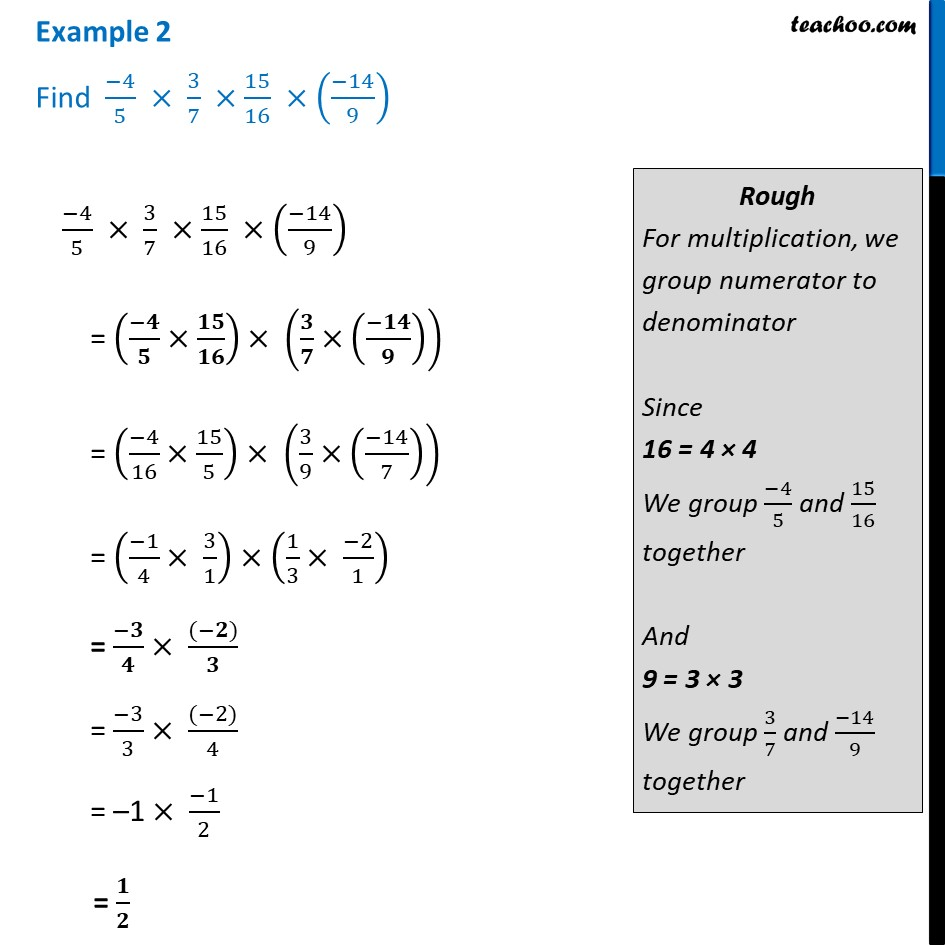Examples

Chapter 1 Class 8 Rational Numbers
Serial order wiseLearn in your speed, with individual attention - Teachoo Maths 1-on-1 Class

### Transcript

Example 2 Find (−4)/5 × 3/7 ×15/16 ×((−14)/9) (−4)/5 × 3/7 ×15/16 ×((−14)/9) = ((−𝟒)/𝟓×𝟏𝟓/𝟏𝟔) × (𝟑/𝟕×((−𝟏𝟒)/𝟗)) = ((−4)/16×15/5) × (3/9×((−14)/7)) = ((−1)/4× 3/1)×(1/3× (−2)/1) = (−𝟑)/𝟒 × ((−𝟐))/𝟑 = (−3)/3 × ((−2))/4 = –1 × (−1)/2 = 𝟏/𝟐 Rough For multiplication, we group numerator to denominator Since 16 = 4 × 4 We group (−4)/5 and 15/16 together And 9 = 3 × 3 We group 3/7 and (−14)/9 together